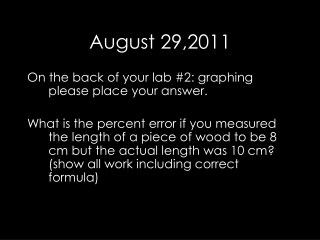DownloadDownload PresentationAugust 29,2011

# August 29,2011

Télécharger la présentation## August 29,2011

- - - - - - - - - - - - - - - - - - - - - - - - - - - E N D - - - - - - - - - - - - - - - - - - - - - - - - - - -
##### Presentation Transcript

1. August 29,2011 On the back of your lab #2: graphing please place your answer. What is the percent error if you measured the length of a piece of wood to be 8 cm but the actual length was 10 cm? (show all work including correct formula)

2. Objective Convert metric units from one to another Use various equipment to measure items Agenda Metric notes/ worksheet Lab #3: measurement HW: Finish Lab #3 Reminder: Lab #2: Graphing is due by the end of the day

3. Metric System Basics

4. Metric System • The metric system is based on a base unit that corresponds to a certain kind of measurement • Length = meter • Volume = liter • Weight (Mass) = gram • Prefixes plus base units make up the metric system • Example: • Centi + meter = Centimeter • Kilo + liter = Kiloliter

5. Metric System • The three prefixes that we will use the most are: • kilo • centi • milli

6. Metric System • So if you needed to measure length you would choose meter as your base unit • Length of a tree branch • 1.5 meters • Length of a room • 5 meters • Length of a ball of twine stretched out • 25 meters

7. Metric System • But what if you need to measure a longer distance, like from your house to school? • Let’s say you live approximately 10 miles from school • 10 miles = 16093 meters • 16093 is a big number, but what if you could add a prefix onto the base unit to make it easier to manage: • 16093 meters = 16.093 kilometers (or 16.1 if rounded to 1 decimal place)

8. Metric System • These prefixes are based on powers of 10. What does this mean? • From each prefix every “step” is either: • 10 times larger or • 10 times smaller • For example • Centimeters are 10 times larger than millimeters • 1 centimeter = 10 millimeters

9. Metric System • Centimeters are 10 times larger than millimeters so it takes more millimeters for the same length 1 centimeter = 10 millimeters Example not to scale 40 41 40 41 1 cm

10. Metric System • For each “step” to right, you are multiplying by 10 • For example, let’s go from a base unit to centi 1 liter = 10 deciliters = 100 centiliters 2 grams = 20 decigrams = 200 centigrams ( 1 x 10 = 10) = (10 x 10 = 100) (2 x 10 = 20) = (20 x 10 = 200)

11. Metric System • An easy way to move within the metric system is by moving the decimal point one place for each “step” desired Example: change meters to centimeters 1 meter = 10 decimeters = 100 centimeters or 1.00 meter = 10.0 decimeters = 100. centimeters

12. Metric System • Now let’s try our previous example from meters to kilometers: 16093 meters = 1609.3 decameters = 160.93 hectometers = 16.093 kilometers • So for every “step” from the base unit to kilo, we moved the decimal 1 place to the left (the same direction as in the diagram below)

13. Metric System • If you move to the left in the diagram, move the decimal to the left • If you move to the right in the diagram, move the decimal to the right

14. Metric System • Now let’s start from centimeters and convert to kilometers 400000 centimeters = 4 kilometers 400000 centimeters = 4.00000 kilometers

15. Metric System • Now let’s start from meters and convert to kilometers 4000 meters = 4 kilometers • Now let’s start from centimeters and convert to meters • 4000 centimeters = 40 meters

16. Metric System • Now let’s start from meters and convert to centimeters 5 meters = 500 centimeters • Now let’s start from kilometers and convert to meters • .3 kilometers = 300 meters

17. Metric System • Now let’s start from kilometers and convert to millimeters 4 kilometers = 4000000 millimeters or 4 kilometers = 40 hectometers = 400 decameters = 4000 meters = 40000 decimeters = 400000 centimeters = 4000000 millimeters

18. Metric System • Summary • Base units in the metric system are meter, liter, gram • Metric system is based on powers of 10 • For conversions within the metric system, each “step” is 1 decimal place to the right or left • Using the diagram below, converting to the right, moves the decimal to the right and vice versa

19. Metric Mania • Let’s practice converting in the metric system

20. Lab #3: Measurement • Objective: • To learn how to use various measuring devices

21. Ticket out the door • On a piece of paper write down how you use measurements each day.